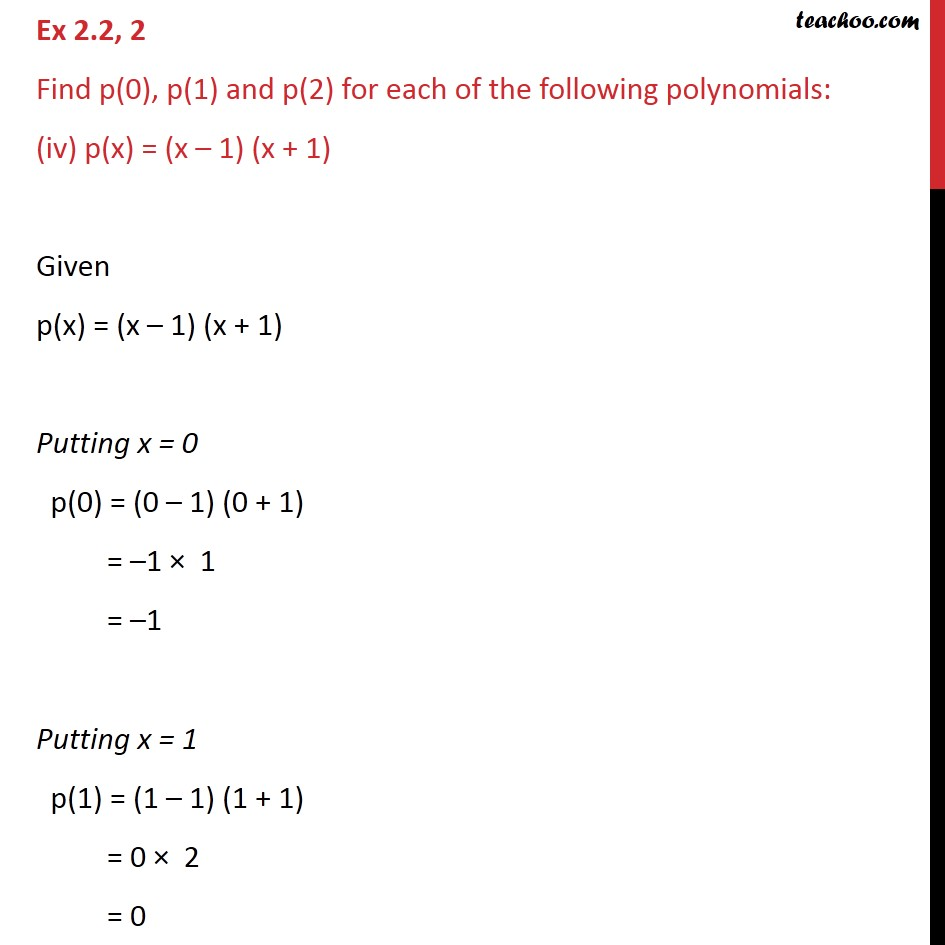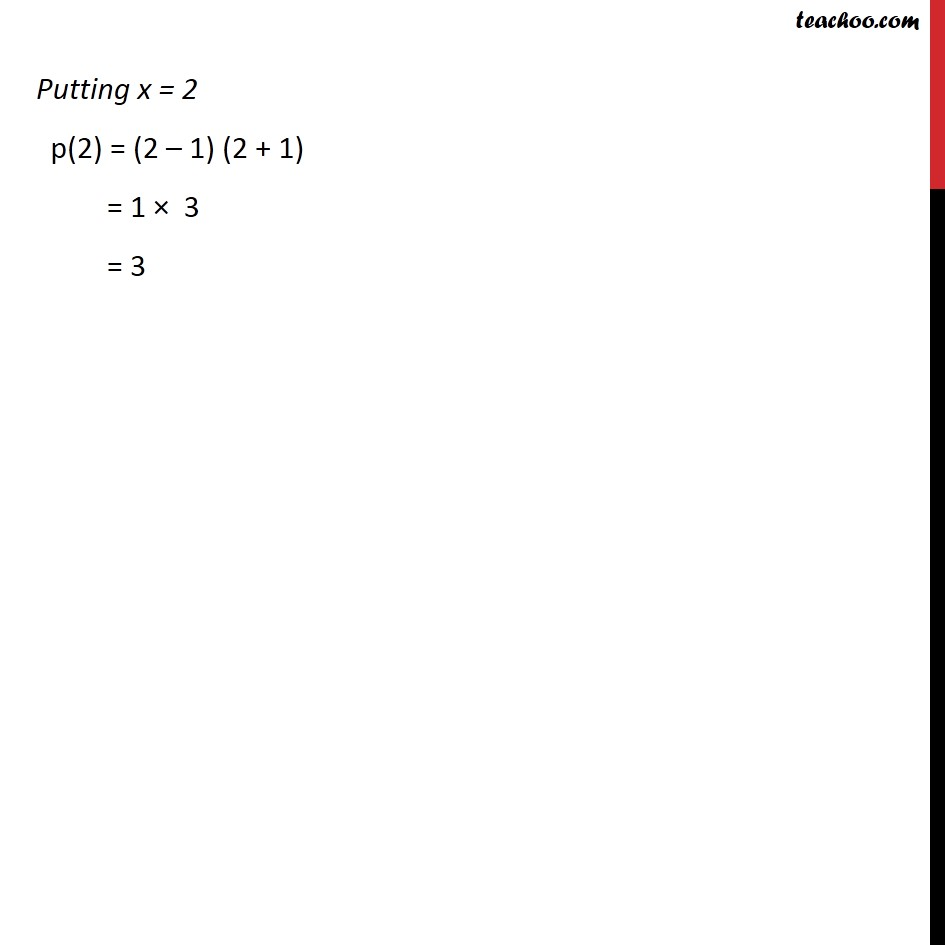Ex 2.2

Chapter 2 Class 9 Polynomials
Serial order wiseGet live Maths 1-on-1 Classs - Class 6 to 12

### Transcript

Ex 2.2, 2 Find p(0), p(1) and p(2) for each of the following polynomials: (iv) p(x) = (x – 1) (x + 1) Given p(x) = (x – 1) (x + 1) Putting x = 0 p(0) = (0 – 1) (0 + 1) = –1 × 1 = –1 Putting x = 1 p(1) = (1 – 1) (1 + 1) = 0 × 2 = 0 Putting x = 2 p(2) = (2 – 1) (2 + 1) = 1 × 3 = 3